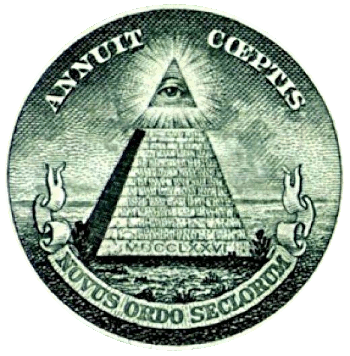### Top Ten Numbers

September 5, 2012

Top ten lists are always contentious. In lists of the top ten movies of all time, Gone with the Wind, Casablanca and Citizen Kane are typically there, but after those it's a hodge podge of various tastes. The Latin saying, "De gustibus non dispudandum est" ("About taste there can be no argument"), is apt. One man's Citizen Kane is another man's Teenage Mutant Ninja Turtles.

The American Mathematical Society web site has a page that lists recent articles about mathematics in the popular media. One interesting item on the most recent list was a Business Insider article about "The 17 Equations That Changed The World." The equations were presented in a 2012 book, "In Pursuit of the Unknown: 17 Equations That Changed the World," by Ian Stewart.

The interesting thing about this list is that only half of the equations are purely mathematical (e.g., the Pythagorean equation). The others are equations that express physical laws, such as Newton's law of universal gravitation. One world-changing equation on the list is the Black–Scholes derivative equation. Yes, it did change the world; but, perhaps, not for the better.

This list of equations refers to another article that was just as interesting, "The 10 Most Important Numbers In The World." Here are the top ten numbers, according to Ben Duronio and Walter Hickey of the Business Insider:
 • Pi (π), also known as Archimedes' Constant, 3.14159265... • e, also known as Euler's Constant, 2.718281828... • The Golden Ratio (φ), 1.6180339887... • Planck Constant, 6.62606957 x 10−34 joule-second • Avogadro Constant: 6.0221515 x 1023 mole-1 • The speed of light, 299,792,458 meters per second • Gravitational Constant (G): 6.6738 x 10-11 m3 kg-1 s-2 • Boltzmann's Constant, 1.3806488 x 10−23 joule-kelvin-1 • Imaginary Unit (i = √-1) • Euler's Identity, ei pi + 1 = 0
The last item, wonderful as it is in combining e, i, π, 1 and 0, should really have been on the equation list, so that gives us nine numbers. The purists will claim that only four numbers on the list are really numbers, since these are the only dimensionless numbers there, but the list is intended as an expression of a layman's idea of a number.Archimedes in his bath, by Johann Petrejus, 1547.

Archimedes is known for pi, among many other things. How many circles, or circular arcs, can you find in this image?

(Via Wikimedia Commons))

All these numbers are important to life as we know it, but what of others not on the list? Zero is an important number, important enough to be the topic of an entire book, "Zero: The Biography of a Dangerous Idea," by Charles Seife. Peripherally, it did make it on this list in Euler's identity.

If these nine (or ten) numbers have piqued your interest, I refer you to a previous article (Special Numbers, September 21, 2011). The article recites some special properties of certain numbers numbers. For example,
38 is the last Roman numeral when written lexicographically (alphabetically), XXXVIII.

206 is the smallest number whose English name contains all five vowels exactly once (Two hundred and six). If you allow the "sometimes y" criterion, it would be two hundred and sixty.

428 has the property that its square, 183184, is the concatenation of two consecutive numbers.

9996 has a square, 99920016, formed by inserting a block of digits inside itself.Another example of Roman numerals.

The Great Seal of the United States, from a US dollar bill.

MDCCLXXVI = 1776.

(Via Wikimedia Commons))### References:

Linked Keywords: Film; movie; Gone with the Wind; Casablanca; Citizen Kane; hodge podge; Latin; saying; De gustibus non dispudandum est; Teenage Mutant Ninja Turtles; American Mathematical Society; mathematics; mass media; popular media<; Business Insider; equation; Ian Stewart; Pythagorean equation; physical law; Newton's law of universal gravitation; Black–Scholes derivative equation; number; Pi; Archimedes; e; Leonhard Euler; Golden Ratio; Planck Constant; joule; second; Avogadro Constant; mole; speed of light; meters per second; Gravitational Constant; kilogram; kg; Boltzmann's Constant; kelvin; imaginary unit; Euler's Identity; dimensionless number; layperson; layman; circle; circular arc; Wikimedia Commons; zero; Charles Seife; Roman numeral; lexicographical order; English language; vowel; square; concatenation; consecutive number; digit; Great Seal of the United States; US dollar bill.